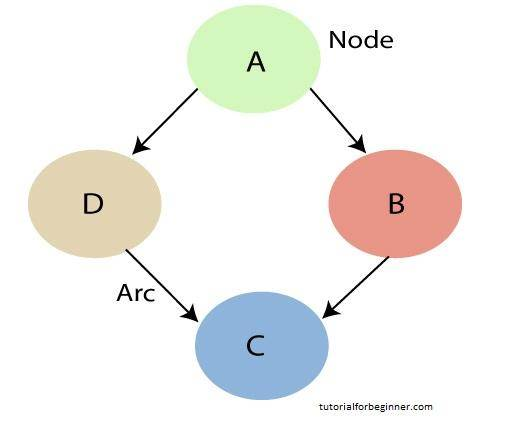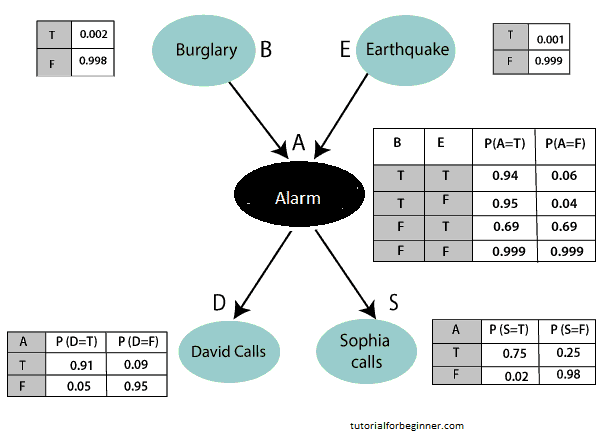# Bayesian Belief Network in artificial intelligence

In this page we will learn about What is Bayesian Belief Network in artificial intelligence?, Joint probability distribution, Explanation of Bayesian network, The semantics of Bayesian Network.

## What is Bayesian Belief Network in artificial intelligence?

The Bayesian belief network is a crucial computer technique for coping with unpredictable events and solving problems. A Bayesian network is defined as:
"A Bayesian network is a probabilistic graphical model which represents a set of variables and their conditional dependencies using a directed acyclic graph."
A Bayesian model is also known as a Bayes network, belief network, or decision network.
Because Bayesian networks are created from a probability distribution and use probability theory for prediction and anomaly detection, they are probabilistic.

Real-world applications are probabilistic in nature, and we require a Bayesian network to express the link between various events. It can also be utilized for tasks like prediction, anomaly detection, diagnostics, automated insight, reasoning, time series prediction, and decision making in uncertain situations.

The Bayesian Network is made up of two pieces that can be used to create models from data and expert opinions:

• Directed Acyclic Graph
• Table of conditional probabilities.

An Influence diagram is a generalized type of Bayesian network that illustrates and solves decision problems under uncertain knowledge.

Nodes and Arcs (directed linkages) make form a Bayesian network graph, where:Each node represents a random variable, which can be either continuous or discontinuous.
The causal relationship or conditional probabilities between random variables are represented by arcs or directed arrows. These directed links, also known as arrows, connect the graph's two nodes.

• In the above diagram, A, B, C, and D are random variables represented by the nodes of the network graph.
• If we are considering node B, which is connected with node A by a directed arrow, then node A is called the parent of Node B.
• Node C is independent of node A.

Note: There are no circular graphs in the Bayesian network graph. As a result, it's referred to as a directed acyclic graph, or DAG.

The Bayesian network is made up of two primary parts.

• Causal Component
• Actual numbers

The influence of the parent on each node in the Bayesian network is determined by the condition probability distribution P(Xi | Parent(Xi)).
The Joint Probability Distribution and Conditional Probability are the foundations of the Bayesian network. So, first, let's look at the joint probability distribution:

## Joint probability distribution:

If we have variables x1, x2, x3,....., xn, then the probabilities of a different combination of x1, x2, x3.. xn, are known as Joint probability distribution.
P[x1, x2, x3,....., xn], it can be written as the following way in terms of the joint probability distribution.

In general for each variable Xi, we can write the equation as:

``` P(Xi|Xi-1,........., X1) = P(Xi |Parents(Xi )) ```

## Explanation of Bayesian network:

Let's look at an example of a Bayesian network by making a directed acyclic graph:

Example: To detect burglary, Harry put a new burglar alarm at his home. The alarm is not only capable of detecting a burglary, but it can also detect mild earthquakes. Harry has two next-door neighbors, David and Sophia, who have agreed to notify Harry at work if they hear the alarm. When David hears the alarm, he always phones Harry, but sometimes he gets confused with the phone ringing and calls at that time as well. Sophia, on the other hand, enjoys listening to loud music and occasionally misses the alarm. We'd like to calculate the likelihood of a burglary alarm in this case.

Calculate the probability that alarm has sounded, but there is neither a burglary, nor an earthquake occurred, and David and Sophia both called the Harry.

### Solution:

• The Bayesian network for the problem described above is shown below. The network topology shows that burglary and earthquake are the parent nodes of the alarm, affecting the probability of the alarm going off directly, but David and Sophia's calls are dependent on the probability of the alarm going off.
• Our assumptions, according to the network, do not directly sense the burglary, do not notice the tiny earthquake, and do not communicate before contacting.
• Conditional probabilities tables, or CPTs, are used to represent the conditional distributions for each node.
• Because all of the entries in the table indicate an exhaustive set of cases for the variable, each row in the CPT must add to 1.
• 2K probabilities are included in a boolean variable with k boolean parents in CPT. As a result, if there are two parents, CPT will have four probability values.

List of all events occurring in this network:

• Burglary (B)
• Earthquake(E)
• Alarm(A)
• David Calls(D)
• Sophia calls(S)

We can express the occurrences in the problem statement as probability: Using joint probability distribution, P[D, S, A, B, E] may rewrite the preceding probability statement:

P[D, S, A, B, E]= P[D | S, A, B, E]. P[S, A, B, E]
= P[D | S, A, B, E]. P[S | A, B, E]. P[A, B, E]
= P [D| A]. P [ S| A, B, E]. P[ A, B, E]
= P[D | A]. P[ S | A]. P[A| B, E]. P[B, E]
= P[D | A ]. P[S | A]. P[A| B, E]. P[B |E]. P[E]Let's take the observed probability for the Burglary and earthquake component:
P(B= True) = 0.002, which is the probability of burglary.
P(B= False)= 0.998, which is the probability of no burglary.
P(E= True)= 0.001, which is the probability of a minor earthquake
P(E= False)= 0.999, Which is the probability that an earthquake not occurred.

We can provide the conditional probabilities as per the below tables:

Conditional probability table for Alarm A:

The Conditional probability of Alarm A depends on Burglar and earthquake:

B E P(A=True) P(A=False)
True True 0.94 0.06
True False 0.95 0.04
False True 0.31 0.69
False False 0.001 0.999

Conditional probability table for David Calls:

David's conditional probability of calling is determined by the probability of Alarm.

A P(D= True) P(D= False)
True 0.91 0.09
False 0.05 0.95

Conditional probability table for Sophia Calls:

Sophia's conditional chance of calling is determined by its Parent Node "Alarm."

A P(S= True) P(S= False)
True 0.75 0.25
False 0.02 0.98

We may formulate the issue statement in the form of a probability distribution using the joint distribution formula:

P(S, D, A, ¬B, ¬E) = P (S|A) *P (D|A)*P (A|¬B ^ ¬E) *P (¬B) *P (¬E).

= 0.75* 0.91* 0.001* 0.998*0.999

= 0.00068045.

Therefore, a Bayesian network can answer any query about the domain by using Joint distribution.

## The semantics of Bayesian Network:

The semantics of the Bayesian network can be understood in two ways, as shown below:

1. To understand the network as the representation of the Joint probability distribution.

It is useful to understand how to construct the network.

2. To understand the network as an encoding of a collection of conditional independence statements.

It is useful in designing inference procedure.